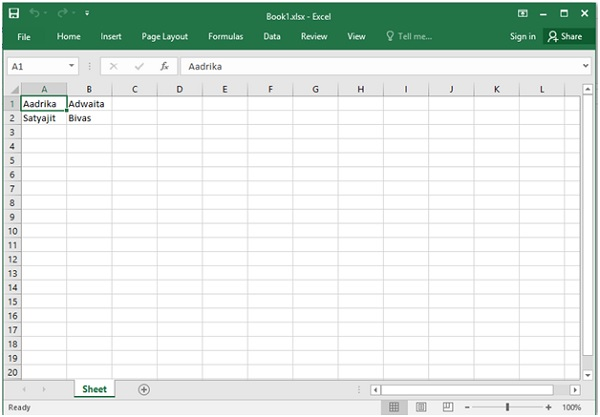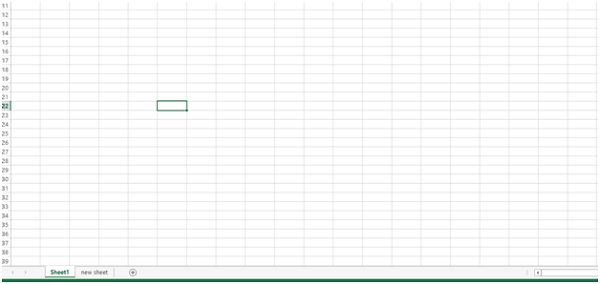# Read and Write to an excel file using Python openpyxl module

Python provides openpyxl module for operating with Excel files.

How to create Excel files, how to write, read etc. can be implemented by this module.

For installing openpyxl module, we can write this command in command prompt

pip install openpyxl


## Example code

import openpyxl
my_wb = openpyxl.Workbook()
my_sheet = my_wb.active
my_sheet_title = my_sheet.title
print("My sheet title: " + my_sheet_title)


## Output

My sheet title:Sheet


## Example code

import openpyxl
my_wb = openpyxl.Workbook()
my_sheet = my_wb.active
my_sheet.title = "My New Sheet"
print("sheet name is : " + sheet.title)


## Output

sheet name is : My New Sheet


## Example code

import openpyxl
my_wb = openpyxl.Workbook()
my_sheet = my_wb.active
c1 = my_sheet.cell(row = 1, column = 1)
c2 = my_sheet.cell(row= 1 , column = 2)
c3 = my_sheet['A2']
c3.value = "Satyajit"
# B2 = column = 2 & row = 2.
c4 = my_sheet['B2']
c4.value = "Bivas"
my_wb.save("C:\Users\TP\Desktop\Book1.xlsx")


## Output## Example code

import openpyxl
my_wb = openpyxl.Workbook()
my_sheet = my_wb.active
my_wb.create_sheet(index = 1 , title = "new sheet")
my_wb.save("C:\Users\TP\Desktop\Book1.xlsx")


## Output## Example code

import openpyxl
my_path = "C:\Users\TP\Desktop\Book1.xlsx"
my_sheet_obj = my_wb_obj.active
print(my_sheet_obj.max_row)


## Output

2


## Example code

import openpyxl
# Give the location of the file
My_path = "C:\Users\TP\Desktop\Book1.xlsx"
my_sheet_obj = my_wb_obj.active
my_cell_obj = my_sheet_obj.cell(row = 1, column = 1)
print(my_cell_obj.value)


## Output

Aadrika


## Example code

import openpyxl
# Give the location of the file
My_path = "C:\Users\TP\Desktop\Book1.xlsx"
my_sheet_obj = my_wb_obj.active
print(sheet_obj.max_column)


## Output

2


## Example code

import openpyxl
# Give the location of the file
my_path = "C:\Users\TP\Desktop\Book1.xlsx"
# workbook object is created
my_sheet_obj = my_wb_obj.active
my_max_col = my_sheet_obj.max_column
for i in range(1, my_max_col + 1):
my_cell_obj = my_sheet_obj.cell(row = 1, column = i)
print(my_cell_obj.value)


## Output

Aadrika Adwaita


## Example code

import openpyxl
# Give the location of the file
my_path = "C:\Users\TP\Desktop\Book1.xlsx"
my_sheet_obj = my_wb_obj.active
my_row = my_sheet_obj.max_row
for i in range(1, my_row + 1):
cell_obj = my_sheet_obj.cell(row = i, column = 1)
print(cell_obj.value)


## Output

Aadrika
Satyajit


## Example code

import openpyxl
# Give the location of the file
my_path = "C:\Users\TP\Desktop\Book1.xlsx"

Satyajit Bivas